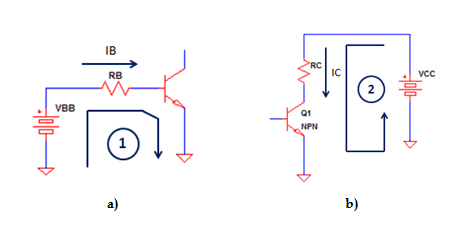# Complete Analysis of a Fixed Bias Circuit using NPN Transistor

This article will uncover the complete analysis of a fixed bias circuit. Figure 73 below shows a simple common emitter configuration. It has a base resistor RB, collector resistor RC but no emitter resistor. In general, this biasing technique is called as non-emitter stabilized bias because there is no emitter resistor. By some, this is also called as the fixed bias.Figure 73 – Non-emitter stabilized circuit. Sometimes this bias technique is called as the fixed bias.

Base-Emitter Analysis

To start the analysis, let’s consider the base-emitter loop (Loop 1) depicted in Figure 74a). We will perform KVL starting from VBBThe first requirement to turn on a bipolar junction transistor is to satisfy its base-emitter voltage requirement. So, it is obvious that the level of VBB must be greater than the  of the transistor which is typically 0.7V for a silicon made while 0.3V for a germanium one.

Collector-Emitter Analysis

Now, we will do KVL on the collector-emitter loop (Loop 2) starting from the VCCThe collector current is a function of the base current and the device beta, so we can write it asAbove equation is only valid as long as the operation is within the active region. At the active region, the collector current of the circuit is not a function of the collector resistor. Changing the value of the resistor will give the same collector current considering the same base current. However, a change in the collector resistor will change the value of collector-emitter voltage described in [Eq. 116]. The change in the VCE has a big effect in the operation of the circuit.

Notes:

VBE is the voltage on the base-emitter junction. It can be stated as the voltage on the base minus the voltage on the emitter (VBE=VB-VE). VCE is the voltage across the emitter-collector. It can also be expressed as the voltage of the collector minus the voltage of the emitter (VCE=VC-VE).

Power Dissipation

The power dissipation of a transistor is the sum of the base-emitter and the collector-emitter dissipations. So for Figure 73, the power dissipation of the base-emitter circuit is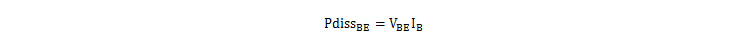While the power dissipation of the collector-emitter section isThe total power dissipation will beOperation at Saturation

When the circuit saturates, the collector current will settle to its maximum level which is dictated by the output circuitry or the collector-emitter loop. For Figure 73, the saturation collector current is(Where  VCEsat is the collector-emitter saturation voltage which is defined in the transistor datasheet.)

Measuring Actual Voltages using a Volt Meter

If we talk about VCE , it is the voltage across the collector-emitter section. When measuring it using a volt meter, the positive lead of the meter should be connected in the collector while the negative lead must be in the emitter as shown in Figure 75.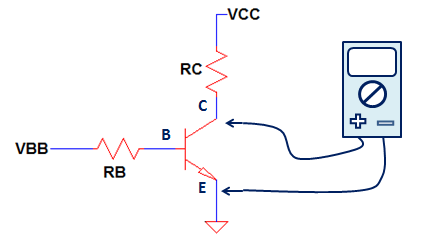Figure 75 – Multi meter lead setup when measuring the voltage across the collector-emitter. VCE is the differential voltage of the collector and the emitter leads. The voltmeter leads should be connected to the respected terminal such that the positive lead to the collector whiles the negative one to the emitter. In this example, the negative lead is can be connected to the circuit ground directly because there is no emitter resistor which makes the emitter voltage the same level with the ground. For circuits having a resistor in the emitter, the negative lead should be on the emitter to get the real collector-emitter voltage.

Figure 76 shows the volt meter lead arrangement when measuring the voltage across base-emitter junction and the voltage drop of the base resistor RB . As a general rule, always insert or tap the positive lead of the measuring device to the section where higher potential is expected and the negative lead to the lower potential.

Example 21

Solve for the following using the circuit in Figure 77.
a) Base current,
b) Collector current
c) VCE
d) VC
e) VB
f) VBC
g) Power Dissipation

Solution

a) Solving base current using [Eq. 115]b) Solving collector current using [Eq. 117]d) The collector voltage is can be solved using the expression as belowThe collector-emitter voltage is already available and the value of the emitter voltage is just zero since emitter is connected directly to the circuit ground. Thus,e) is likewise can be derived from the expression of as belowThe value of  VBE is not defined in the problem so it is by default 0.7V as silicon is the widely used material for transistor. As mentioned above, the value of emitter voltage is zero since it is directly connected to the circuit ground. Therefore,f) The voltage is the difference of the base and the collector voltage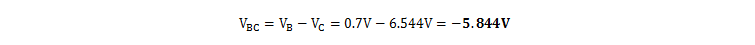The negative voltage means that the base-collector junction is reversed biased. This is really expected because the circuit is operating in the active region. As defined earlier, at active region the base-emitter junction is forward biased while the base-collector is reversed biased.

g) The power dissipation of the transistor is the sum of the base-emitter and the collector-emitter dissipations. Using [Eq. 118]Checking for Beta Variation Effect

The major contributor for the operation shift or Q-point change of a transistor circuit is the big variation of the transistor current gain, β. For instance, a BC817-25 transistor has a beta which is ranging from 160 to 400. This is a huge range and surely changes the operating point of the circuit if not properly designed.

With the same circuit in Figure 77, let us solve for the a) base current, b) collector current and c) Collector-emitter voltage but this time doubling the beta.

a) Solving base current using [Eq. 115]b) Solving collector current using [Eq. 117]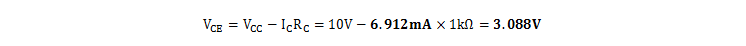As you notice, the base current is the same because in the first place beta is not a factor of it. The collector current has been doubled in value and the collector-emitter voltage is less than half the original value. The operation of the circuit is very affected by doubling the current gain. If you are designing a critical application, this bias type is not a good choice.

To summarize, the complete analysis of a fixed bias circuit is can be done by doing KVL in the base-emitter and collector-emitter loop.

This site uses Akismet to reduce spam. Learn how your comment data is processed.